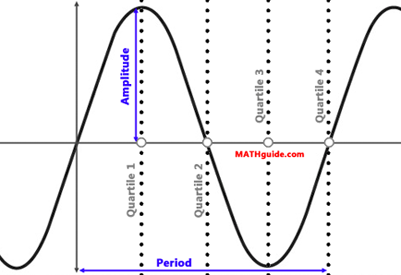Graphing Sine & Cosine: Amplitude & Period
View the Lesson | MATHguide homepage Updated August 6th, 2021Problem: f(x) = 2 sin 3 x. On a sheet of paper, graph the function in degrees. Determine the function's y-intercept, amplitude, interval, period, and the four x-values that mark the location of its quartiles. Responses: y-intercept = (, )    amplitude =    interval = [, ]    period =    quartile 1 =    quartile 2 =    quartile 3 =    quartile 4 =